# Mean State

Period Mean (original grids) [W/m2]
Model Period Mean (intersection) [W/m2]
Model Period Mean (complement) [W/m2]
Benchmark Period Mean (intersection) [W/m2]
Benchmark Period Mean (complement) [W/m2]
Bias [W/m2]
RMSE [W/m2]
Phase Shift [months]
Bias Score 
RMSE Score 
Seasonal Cycle Score 
Spatial Distribution Score 
Interannual Variability Score 
Overall Score 
Benchmark [-] 457.
CLM4 [-] 458. 458. 456. 465. 2.39 14.9 1.26 0.470 0.380 0.823 0.938 0.634 0.604
CLM4.5 [-] 462. 462. 456. 465. 6.40 16.3 1.35 0.428 0.383 0.808 0.957 0.635 0.599
CLM5 [-] 463. 463. 456. 465. 7.83 16.4 1.44 0.460 0.382 0.805 0.979 0.612 0.603
Period Mean (original grids) [W/m2]
Model Period Mean (intersection) [W/m2]
Model Period Mean (complement) [W/m2]
Benchmark Period Mean (intersection) [W/m2]
Benchmark Period Mean (complement) [W/m2]
Bias [W/m2]
RMSE [W/m2]
Phase Shift [months]
Bias Score 
RMSE Score 
Seasonal Cycle Score 
Spatial Distribution Score 
Interannual Variability Score 
Overall Score 
Benchmark [-] 466.
CLM4 [-] 476. 477. 467. 458. 10.1 19.4 0.592 0.591 0.498 0.947 0.996 0.671 0.700
CLM4.5 [-] 482. 482. 467. 458. 15.3 22.2 0.529 0.489 0.509 0.954 0.944 0.654 0.676
CLM5 [-] 484. 484. 467. 458. 17.2 23.5 0.637 0.460 0.502 0.942 0.951 0.622 0.663
Period Mean (original grids) [W/m2]
Model Period Mean (intersection) [W/m2]
Model Period Mean (complement) [W/m2]
Benchmark Period Mean (intersection) [W/m2]
Benchmark Period Mean (complement) [W/m2]
Bias [W/m2]
RMSE [W/m2]
Phase Shift [months]
Bias Score 
RMSE Score 
Seasonal Cycle Score 
Spatial Distribution Score 
Interannual Variability Score 
Overall Score 
Benchmark [-] 443.
CLM4 [-] 446. 446. 443. 446. 4.18 16.7 1.08 0.636 0.484 0.857 1.00 0.658 0.687
CLM4.5 [-] 453. 453. 443. 446. 9.71 18.3 1.09 0.557 0.485 0.855 0.998 0.658 0.673
CLM5 [-] 452. 452. 443. 446. 9.13 18.8 1.38 0.570 0.463 0.814 1.00 0.603 0.652
Period Mean (original grids) [W/m2]
Model Period Mean (intersection) [W/m2]
Model Period Mean (complement) [W/m2]
Benchmark Period Mean (intersection) [W/m2]
Benchmark Period Mean (complement) [W/m2]
Bias [W/m2]
RMSE [W/m2]
Phase Shift [months]
Bias Score 
RMSE Score 
Seasonal Cycle Score 
Spatial Distribution Score 
Interannual Variability Score 
Overall Score 
Benchmark [-] 279.
CLM4 [-] 276. 276. 278. 296. 0.256 15.9 0.121 0.911 0.797 0.990 0.995 0.781 0.879
CLM4.5 [-] 275. 276. 278. 296. 0.179 15.2 0.123 0.911 0.806 0.990 0.998 0.764 0.879
CLM5 [-] 277. 278. 278. 296. 2.37 15.4 0.127 0.903 0.808 0.990 1.00 0.744 0.875
Period Mean (original grids) [W/m2]
Model Period Mean (intersection) [W/m2]
Model Period Mean (complement) [W/m2]
Benchmark Period Mean (intersection) [W/m2]
Benchmark Period Mean (complement) [W/m2]
Bias [W/m2]
RMSE [W/m2]
Phase Shift [months]
Bias Score 
RMSE Score 
Seasonal Cycle Score 
Spatial Distribution Score 
Interannual Variability Score 
Overall Score 
Benchmark [-] 457.
CLM4 [-] 452. 452. 454. 467. -0.662 13.6 1.63 0.374 0.371 0.755 0.589 0.602 0.510
CLM4.5 [-] 454. 454. 454. 467. 0.984 14.0 1.68 0.331 0.375 0.741 0.602 0.589 0.502
CLM5 [-] 459. 459. 454. 467. 5.54 14.6 1.55 0.286 0.378 0.766 0.648 0.579 0.506
Period Mean (original grids) [W/m2]
Model Period Mean (intersection) [W/m2]
Model Period Mean (complement) [W/m2]
Benchmark Period Mean (intersection) [W/m2]
Benchmark Period Mean (complement) [W/m2]
Bias [W/m2]
RMSE [W/m2]
Phase Shift [months]
Bias Score 
RMSE Score 
Seasonal Cycle Score 
Spatial Distribution Score 
Interannual Variability Score 
Overall Score 
Benchmark [-] 312.
CLM4 [-] 313. 313. 311. 334. 2.07 10.5 0.0356 0.936 0.879 0.998 0.999 0.875 0.928
CLM4.5 [-] 314. 314. 311. 334. 2.37 10.6 0.0385 0.937 0.879 0.997 0.996 0.874 0.927
CLM5 [-] 317. 317. 311. 334. 5.51 11.6 0.0521 0.909 0.884 0.997 0.999 0.859 0.922
Period Mean (original grids) [W/m2]
Model Period Mean (intersection) [W/m2]
Model Period Mean (complement) [W/m2]
Benchmark Period Mean (intersection) [W/m2]
Benchmark Period Mean (complement) [W/m2]
Bias [W/m2]
RMSE [W/m2]
Phase Shift [months]
Bias Score 
RMSE Score 
Seasonal Cycle Score 
Spatial Distribution Score 
Interannual Variability Score 
Overall Score 
Benchmark [-] 364.
CLM4 [-] 362. 361. 363. 399. 0.496 13.2 0.107 0.873 0.825 0.992 0.993 0.782 0.882
CLM4.5 [-] 362. 362. 363. 399. 0.962 13.2 0.105 0.870 0.827 0.992 0.992 0.776 0.881
CLM5 [-] 366. 365. 363. 399. 4.07 14.2 0.164 0.858 0.822 0.988 0.996 0.762 0.875
Period Mean (original grids) [W/m2]
Model Period Mean (intersection) [W/m2]
Model Period Mean (complement) [W/m2]
Benchmark Period Mean (intersection) [W/m2]
Benchmark Period Mean (complement) [W/m2]
Bias [W/m2]
RMSE [W/m2]
Phase Shift [months]
Bias Score 
RMSE Score 
Seasonal Cycle Score 
Spatial Distribution Score 
Interannual Variability Score 
Overall Score 
Benchmark [-] 448.
CLM4 [-] 447. 448. 446. 467. 3.72 16.5 0.643 0.661 0.562 0.936 0.988 0.680 0.732
CLM4.5 [-] 452. 452. 446. 467. 7.61 18.1 0.659 0.614 0.567 0.933 0.986 0.671 0.723
CLM5 [-] 453. 454. 446. 467. 9.50 18.9 0.825 0.605 0.557 0.913 0.979 0.628 0.707
Period Mean (original grids) [W/m2]
Model Period Mean (intersection) [W/m2]
Model Period Mean (complement) [W/m2]
Benchmark Period Mean (intersection) [W/m2]
Benchmark Period Mean (complement) [W/m2]
Bias [W/m2]
RMSE [W/m2]
Phase Shift [months]
Bias Score 
RMSE Score 
Seasonal Cycle Score 
Spatial Distribution Score 
Interannual Variability Score 
Overall Score 
Benchmark [-] 281.
CLM4 [-] 282. 282. 281. 288. 2.23 14.4 0.0850 0.930 0.802 0.993 1.00 0.788 0.886
CLM4.5 [-] 281. 282. 281. 288. 1.72 12.7 0.0824 0.936 0.821 0.993 1.00 0.769 0.890
CLM5 [-] 284. 284. 281. 288. 4.17 13.2 0.0840 0.914 0.827 0.993 1.00 0.767 0.888
Period Mean (original grids) [W/m2]
Model Period Mean (intersection) [W/m2]
Model Period Mean (complement) [W/m2]
Benchmark Period Mean (intersection) [W/m2]
Benchmark Period Mean (complement) [W/m2]
Bias [W/m2]
RMSE [W/m2]
Phase Shift [months]
Bias Score 
RMSE Score 
Seasonal Cycle Score 
Spatial Distribution Score 
Interannual Variability Score 
Overall Score 
Benchmark [-] 441.
CLM4 [-] 447. 447. 443. 426. 1.41 12.4 0.347 0.811 0.703 0.971 0.988 0.780 0.826
CLM4.5 [-] 447. 447. 443. 426. 1.93 12.8 0.354 0.798 0.700 0.969 0.987 0.778 0.822
CLM5 [-] 449. 449. 443. 426. 3.62 13.2 0.381 0.797 0.692 0.969 0.990 0.739 0.813
Period Mean (original grids) [W/m2]
Model Period Mean (intersection) [W/m2]
Model Period Mean (complement) [W/m2]
Benchmark Period Mean (intersection) [W/m2]
Benchmark Period Mean (complement) [W/m2]
Bias [W/m2]
RMSE [W/m2]
Phase Shift [months]
Bias Score 
RMSE Score 
Seasonal Cycle Score 
Spatial Distribution Score 
Interannual Variability Score 
Overall Score 
Benchmark [-] 368.
CLM4 [-] 362. 362. 366. 389. -1.28 12.2 0.248 0.854 0.760 0.983 0.999 0.807 0.861
CLM4.5 [-] 361. 361. 366. 389. -1.80 11.7 0.207 0.844 0.772 0.986 0.999 0.805 0.863
CLM5 [-] 365. 365. 366. 389. 1.41 11.8 0.429 0.859 0.773 0.971 0.999 0.781 0.859
Period Mean (original grids) [W/m2]
Model Period Mean (intersection) [W/m2]
Model Period Mean (complement) [W/m2]
Benchmark Period Mean (intersection) [W/m2]
Benchmark Period Mean (complement) [W/m2]
Bias [W/m2]
RMSE [W/m2]
Phase Shift [months]
Bias Score 
RMSE Score 
Seasonal Cycle Score 
Spatial Distribution Score 
Interannual Variability Score 
Overall Score 
Benchmark [-] 399.
CLM4 [-] 377. 377. 376. 409. 2.37 14.8 0.497 0.760 0.668 0.944 1.00 0.727 0.795
CLM4.5 [-] 378. 378. 376. 409. 3.73 15.1 0.495 0.738 0.672 0.944 1.00 0.723 0.791
CLM5 [-] 381. 381. 376. 409. 6.29 16.1 0.539 0.722 0.667 0.940 0.999 0.692 0.781
Period Mean (original grids) [W/m2]
Model Period Mean (intersection) [W/m2]
Model Period Mean (complement) [W/m2]
Benchmark Period Mean (intersection) [W/m2]
Benchmark Period Mean (complement) [W/m2]
Bias [W/m2]
RMSE [W/m2]
Phase Shift [months]
Bias Score 
RMSE Score 
Seasonal Cycle Score 
Spatial Distribution Score 
Interannual Variability Score 
Overall Score 
Benchmark [-] 450.
CLM4 [-] 451. 450. 450. 446. 0.525 14.7 0.346 0.848 0.763 0.974 1.00 0.741 0.848
CLM4.5 [-] 451. 451. 450. 446. 0.875 14.7 0.336 0.848 0.763 0.975 1.00 0.737 0.847
CLM5 [-] 452. 452. 450. 446. 2.24 15.1 0.358 0.844 0.760 0.973 1.00 0.716 0.842
Period Mean (original grids) [W/m2]
Model Period Mean (intersection) [W/m2]
Model Period Mean (complement) [W/m2]
Benchmark Period Mean (intersection) [W/m2]
Benchmark Period Mean (complement) [W/m2]
Bias [W/m2]
RMSE [W/m2]
Phase Shift [months]
Bias Score 
RMSE Score 
Seasonal Cycle Score 
Spatial Distribution Score 
Interannual Variability Score 
Overall Score 
Benchmark [-] 281.
CLM4 [-] 284. 284. 281. 293. 3.30 14.8 0.172 0.909 0.804 0.986 1.00 0.796 0.883
CLM4.5 [-] 284. 284. 281. 293. 3.03 14.9 0.160 0.912 0.801 0.987 1.00 0.782 0.880
CLM5 [-] 287. 286. 281. 293. 6.06 16.8 0.168 0.888 0.790 0.987 1.00 0.763 0.870
Period Mean (original grids) [W/m2]
Model Period Mean (intersection) [W/m2]
Model Period Mean (complement) [W/m2]
Benchmark Period Mean (intersection) [W/m2]
Benchmark Period Mean (complement) [W/m2]
Bias [W/m2]
RMSE [W/m2]
Phase Shift [months]
Bias Score 
RMSE Score 
Seasonal Cycle Score 
Spatial Distribution Score 
Interannual Variability Score 
Overall Score 
Benchmark [-] 442.
CLM4 [-] 446. 446. 439. 459. 11.0 18.4 0.860 0.520 0.553 0.889 0.990 0.727 0.705
CLM4.5 [-] 446. 446. 439. 459. 10.4 18.3 0.873 0.522 0.552 0.886 0.966 0.725 0.700
CLM5 [-] 447. 447. 439. 459. 12.0 18.6 0.885 0.510 0.561 0.893 0.950 0.672 0.691
Period Mean (original grids) [W/m2]
Model Period Mean (intersection) [W/m2]
Model Period Mean (complement) [W/m2]
Benchmark Period Mean (intersection) [W/m2]
Benchmark Period Mean (complement) [W/m2]
Bias [W/m2]
RMSE [W/m2]
Phase Shift [months]
Bias Score 
RMSE Score 
Seasonal Cycle Score 
Spatial Distribution Score 
Interannual Variability Score 
Overall Score 
Benchmark [-] 386.
CLM4 [-] 385. 385. 384. 429. 3.03 9.72 0.120 0.904 0.849 0.991 1.00 0.882 0.912
CLM4.5 [-] 387. 387. 384. 429. 4.69 10.5 0.0936 0.883 0.853 0.993 0.998 0.880 0.910
CLM5 [-] 391. 391. 384. 429. 8.89 13.0 0.112 0.823 0.856 0.992 0.993 0.853 0.896
Period Mean (original grids) [W/m2]
Model Period Mean (intersection) [W/m2]
Model Period Mean (complement) [W/m2]
Benchmark Period Mean (intersection) [W/m2]
Benchmark Period Mean (complement) [W/m2]
Bias [W/m2]
RMSE [W/m2]
Phase Shift [months]
Bias Score 
RMSE Score 
Seasonal Cycle Score 
Spatial Distribution Score 
Interannual Variability Score 
Overall Score 
Benchmark [-] 324.
CLM4 [-] 325. 325. 323. 347. 2.59 11.7 0.0506 0.909 0.834 0.996 0.976 0.797 0.891
CLM4.5 [-] 325. 325. 323. 347. 2.59 11.8 0.0528 0.910 0.830 0.996 0.982 0.786 0.889
CLM5 [-] 328. 328. 323. 347. 5.87 13.2 0.104 0.881 0.831 0.993 0.972 0.781 0.882
Period Mean (original grids) [W/m2]
Model Period Mean (intersection) [W/m2]
Model Period Mean (complement) [W/m2]
Benchmark Period Mean (intersection) [W/m2]
Benchmark Period Mean (complement) [W/m2]
Bias [W/m2]
RMSE [W/m2]
Phase Shift [months]
Bias Score 
RMSE Score 
Seasonal Cycle Score 
Spatial Distribution Score 
Interannual Variability Score 
Overall Score 
Benchmark [-] 377.
CLM4 [-] 378. 379. 377. 378. 2.19 12.8 0.0869 0.861 0.820 0.994 0.999 0.775 0.878
CLM4.5 [-] 378. 380. 377. 378. 2.51 12.8 0.0852 0.856 0.825 0.994 0.999 0.787 0.881
CLM5 [-] 381. 382. 377. 378. 5.35 14.1 0.110 0.842 0.814 0.992 1.00 0.743 0.867
Period Mean (original grids) [W/m2]
Model Period Mean (intersection) [W/m2]
Model Period Mean (complement) [W/m2]
Benchmark Period Mean (intersection) [W/m2]
Benchmark Period Mean (complement) [W/m2]
Bias [W/m2]
RMSE [W/m2]
Phase Shift [months]
Bias Score 
RMSE Score 
Seasonal Cycle Score 
Spatial Distribution Score 
Interannual Variability Score 
Overall Score 
Benchmark [-] 449.
CLM4 [-] 453. 453. 449. 463. 4.81 12.6 1.19 0.442 0.392 0.842 0.980 0.648 0.616
CLM4.5 [-] 456. 456. 449. 463. 7.39 14.0 1.15 0.361 0.394 0.850 0.973 0.646 0.603
CLM5 [-] 458. 458. 449. 463. 9.09 14.8 1.21 0.318 0.399 0.836 0.991 0.625 0.595
Period Mean (original grids) [W/m2]
Model Period Mean (intersection) [W/m2]
Model Period Mean (complement) [W/m2]
Benchmark Period Mean (intersection) [W/m2]
Benchmark Period Mean (complement) [W/m2]
Bias [W/m2]
RMSE [W/m2]
Phase Shift [months]
Bias Score 
RMSE Score 
Seasonal Cycle Score 
Spatial Distribution Score 
Interannual Variability Score 
Overall Score 
Benchmark [-] 443.
CLM4 [-] 445. 444. 443. 434. 0.689 14.3 0.848 0.705 0.554 0.878 0.985 0.704 0.730
CLM4.5 [-] 447. 447. 443. 434. 3.04 14.5 0.944 0.685 0.556 0.863 0.979 0.714 0.725
CLM5 [-] 449. 449. 443. 434. 5.44 14.2 0.895 0.695 0.564 0.868 0.992 0.663 0.724
Period Mean (original grids) [W/m2]
Model Period Mean (intersection) [W/m2]
Model Period Mean (complement) [W/m2]
Benchmark Period Mean (intersection) [W/m2]
Benchmark Period Mean (complement) [W/m2]
Bias [W/m2]
RMSE [W/m2]
Phase Shift [months]
Bias Score 
RMSE Score 
Seasonal Cycle Score 
Spatial Distribution Score 
Interannual Variability Score 
Overall Score 
Benchmark [-] 383.
CLM4 [-] 384. 383. 381. 396. 2.84 19.9 0.905 0.644 0.567 0.887 0.999 0.659 0.720
CLM4.5 [-] 386. 385. 381. 396. 4.47 20.2 0.914 0.630 0.569 0.886 0.999 0.659 0.719
CLM5 [-] 387. 386. 381. 396. 5.53 20.2 0.891 0.632 0.570 0.890 0.998 0.636 0.716

# Temporally integrated period mean

BENCHMARK MEAN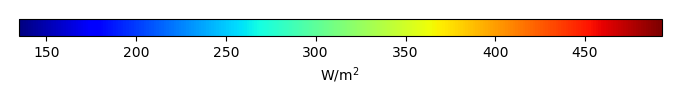MODEL MEANBIAS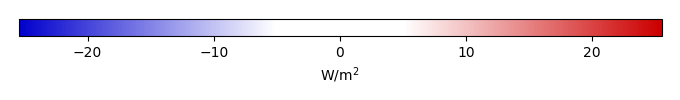BIAS SCORERMSE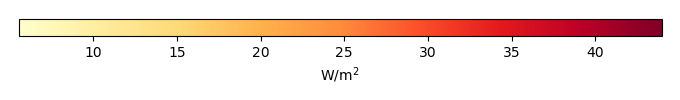RMSE SCOREBENCHMARK INTERANNUAL VARIABILITY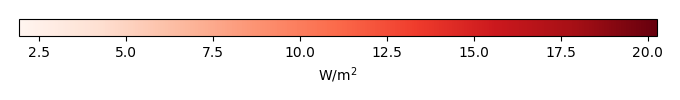MODEL INTERANNUAL VARIABILITYINTERANNUAL VARIABILITY SCOREBENCHMARK MAX MONTHMODEL MAX MONTHDIFFERENCE IN MAX MONTH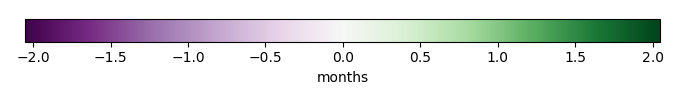SEASONAL CYCLE SCORESPATIAL TAYLOR DIAGRAMMODEL COLORS# Spatially integrated regional mean

MODEL COLORSREGIONAL MEANANNUAL CYCLEMONTHLY ANOMALYANNUAL CYCLE# All Models

BenchmarkCLM4CLM4.5CLM5# Data Information

creation_date: Thu May 8 23:13:11 PDT 2014

source_file: This product is generated from monthly 1 degree CERES EBAF Radiation observations